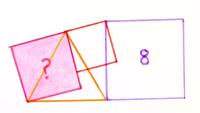# Catriona Agg’s Geometry Puzzles from 2018

1. two rectangles in a semi-circle Dec 31, 2018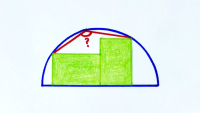2. divided hexagon iii Dec 30, 2018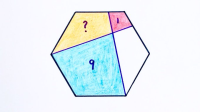3. circles around a triangle Dec 16, 2018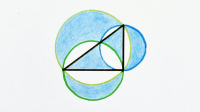4. rectangles stacked in a semi-circle Dec 12, 2018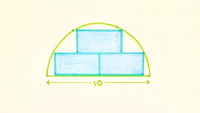5. two circles, two squares Dec 11, 2018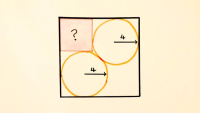6. subdivided square Dec 5, 2018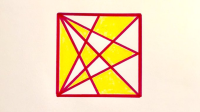7. chords dividing a triangle into five Dec 3, 2018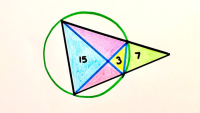8. three rectangles as a triangle Dec 1, 2018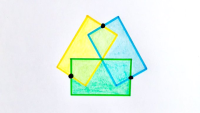9. partitioned square Nov 28, 2018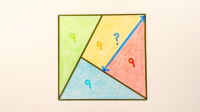10. two squares overlapping a semi-circle Nov 26, 2018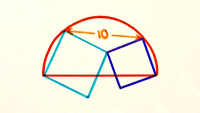11. five triangles in a rectangle Nov 25, 2018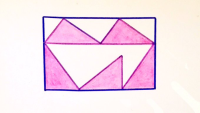12. nested circles and a triangle Nov 24, 2018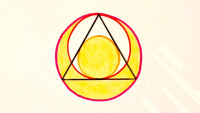13. square in a triangle in a square Nov 23, 2018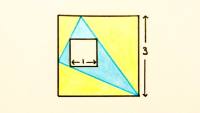14. quarter circles in a rectangle Nov 22, 2018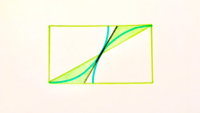15. semi-circles in a quarter circle Nov 21, 2018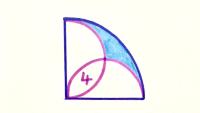16. three squares on a line Nov 19, 2018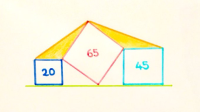17. nested circles and polygons Nov 18, 2018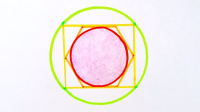18. semi-circle and tangents in a square Nov 17, 2018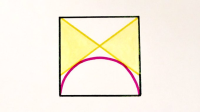19. three regular hexagons ii Nov 14, 2018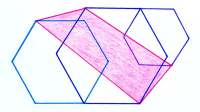20. triangles in a semi-circle Nov 13, 2018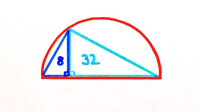21. equilateral triangles in a square Nov 12, 2018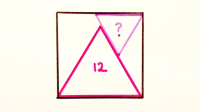22. three aligned triangles Nov 11, 2018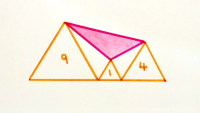23. crossed hexagon Nov 10, 2018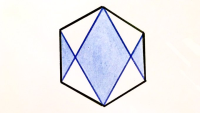24. square in a semi-circle in a square Nov 8, 2018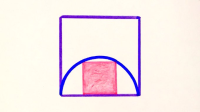25. two equilateral triangles in a semi-circle Nov 7, 2018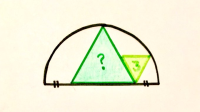26. three squares in a circle Nov 5, 2018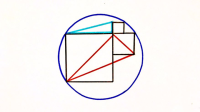27.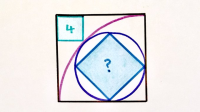28. arcs inside a triangle Nov 2, 2018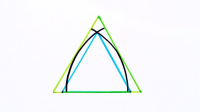29. quadrilateral from two squares in a square Oct 30, 2018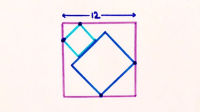30. triangle centres and square corners Oct 29, 2018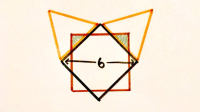31. hexagon and concentric circles Oct 28, 2018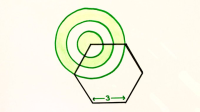32. chord and annulus Oct 27, 2018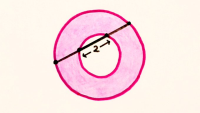33. dissected hexagon Oct 26, 2018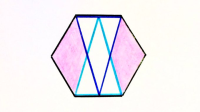34. circles in rhombii sequal Oct 26, 2018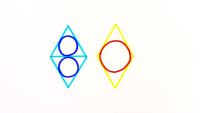35. circles in triangle and rhombus Oct 25, 2018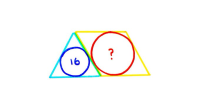36. circles resting on triangles Oct 24, 2018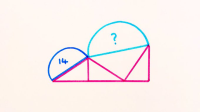37. arbelos Oct 23, 2018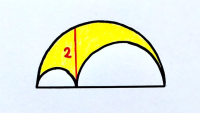38. four circles in a triangle in a circle Oct 22, 2018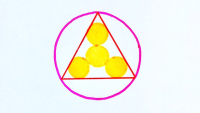39. triangles with a parallelogram Oct 21, 2018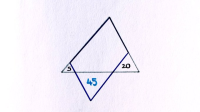40. three squares in a square Oct 18, 2018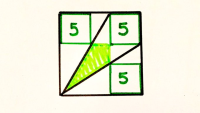41. two circles in an equilateral triangle Oct 17, 2018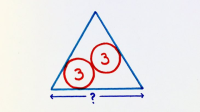42. semi-circles in a square Oct 15, 2018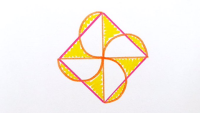43. five rectangles in a square Oct 14, 2018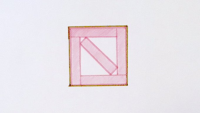44. five overlapping circles Oct 11, 2018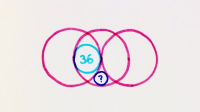45. four regular polygons Oct 8, 2018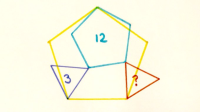46. four squares around a quadrilateral Oct 7, 2018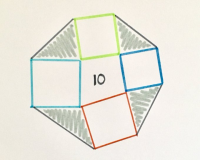47. two perpendicular equilateral triangles Oct 6, 2018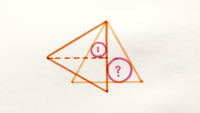48. nested circles and polygons ii Oct 5, 2018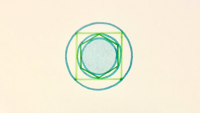49. spiralling semi-circles Oct 3, 2018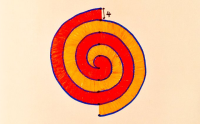50. five decreasing squares Oct 1, 2018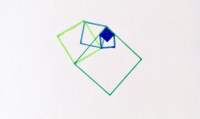51. triangle and semi-circle in a circle Sep 29, 2018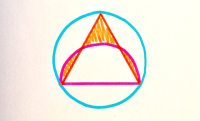52. subdivided parallelogram Sep 27, 2018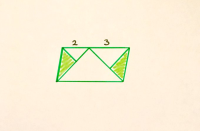53. triangle in four rectangles Sep 26, 2018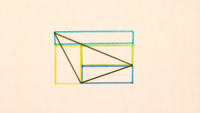54. circle in a trapezium Sep 24, 2018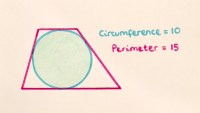55. subdivided triangle Sep 19, 2018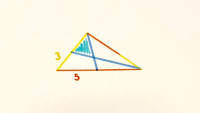56. two circles inside a square Sep 17, 2018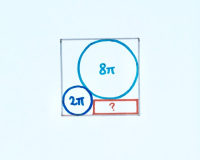57. concentric semi-circles Sep 16, 2018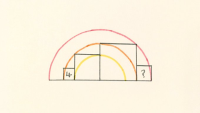58. shaded spikes in a hexagon Sep 14, 2018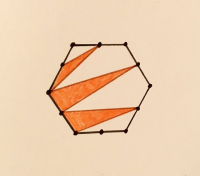59. squares in an octagon Sep 11, 2018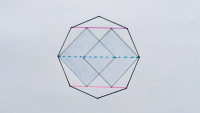60. four squares in a big square Sep 9, 2018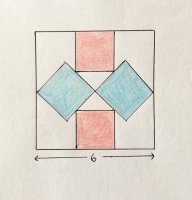61. four stacked squares in a circle in a square Sep 8, 2018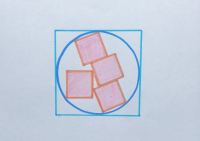62. stacked boxes in circles in squares Sep 8, 2018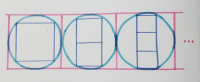63. overlapping square and circle Sep 6, 2018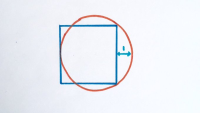64.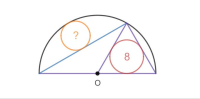65. arcs in a circle Sep 1, 2018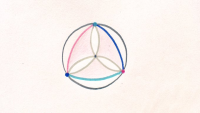66. six squares in a hexagon Aug 31, 2018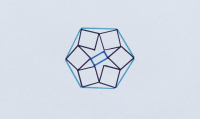67. four equilateral triangles round a square Aug 30, 2018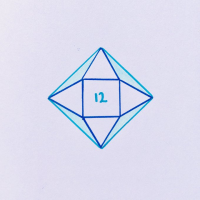68. two overlapping squares iii Aug 28, 2018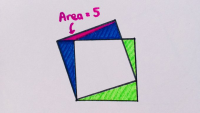69. two isosceles triangles Aug 28, 2018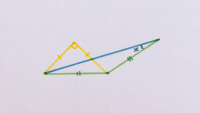70. four isosceles triangles in a circle Aug 26, 2018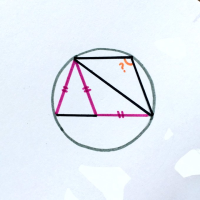71. hexagon in a star in a hexagon Aug 24, 2018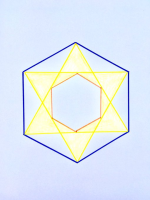72. stacked hexagons Aug 23, 2018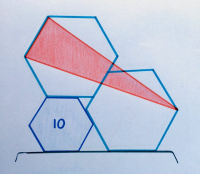73. a square, triangle, and circle in a square Aug 22, 2018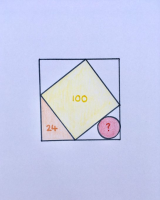74. region of an octagon Aug 21, 2018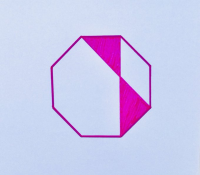75. semi-circle in a square Aug 20, 2018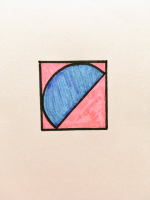76. polygons with equal perimeter Aug 19, 2018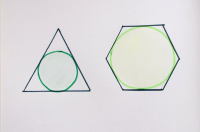77. stacked shapes Aug 19, 2018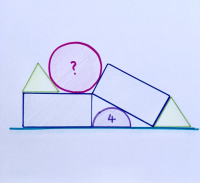78. four squares overlapping a circle Aug 17, 2018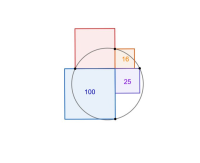79. triangle in circle in triangle in semi-circle Aug 15, 2018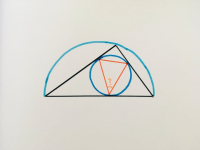80. bell-ringing braids Aug 14, 2018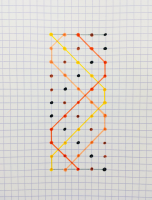81. four equilateral triangles Aug 13, 2018

FourEquilateralTriangles_small.jpg

82. two squares and two equilateral triangles Aug 13, 201883. squares meeting in a circle Aug 12, 2018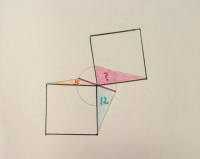84. zig-zag triangles Aug 12, 2018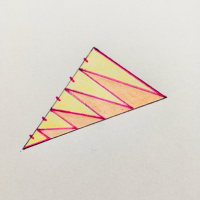85. four aligned squares Aug 10, 2018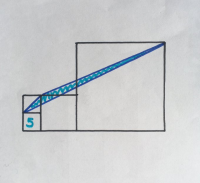86. lengths in a crossed trapezium Aug 9, 2018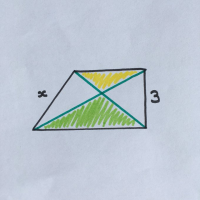87. three aligned squares Aug 9, 2018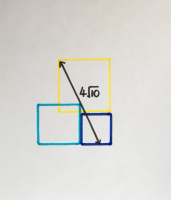88. overlapping squares Aug 8, 2018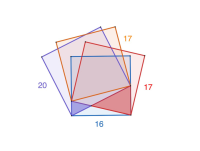89. toppled square Aug 7, 2018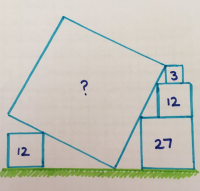90. two rectangles overlapping a circle Aug 2, 2018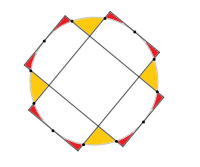91. subdivided circles May 21, 2018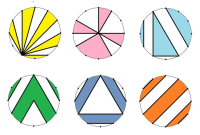92.93.94.95.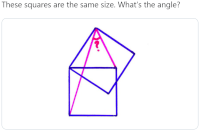96.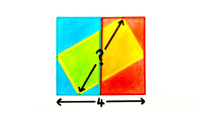97.